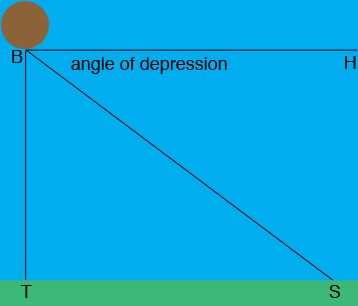SEARCH HOMEMath Central Quandaries & QueriesQuestion from haxir, a student: find the height of the balloon directly above a town if the measure of angle of depression of another town 5km from the first town is 20°!6`Hi Haxir,

Draw a diagram. In my diagram $B$ is the base of the balloon, $T$ is the town below the balloon, $S$ is the second town and $H$ is a point on the horizontal line through $B.$The distance from $T$ to $S$ is 5 km and the angle $HBS$ is the angle of depression which I think has measure $20^o 16'.$ The triangle $BTS$ is a right triangle. What is the measure of the angle $TSB?$ What trig function relates the measure of the angle $TSB$ to the lengths of the sides $BT$ and $TS?$

PennyMath Central is supported by the University of Regina and the Imperial Oil Foundation.